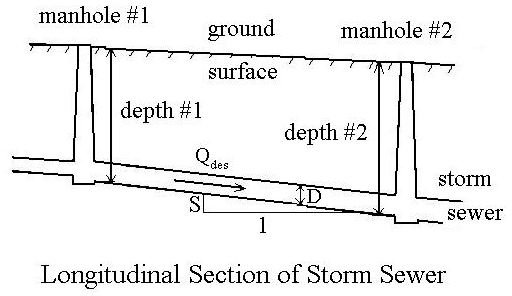# Storm Sewer Design Overview for Good Storm Water Management

Page content

## Introduction

A well designed, functional storm sewer system is an important part of any stormwater drainage system and is crucial to good storm water management. The right hydraulic design gives the proper diameter, slope and depth for a storm sewer line, so that it will drain storm water and not allow it to back up. The criteria to find the proper diameter, slope and depth for a storm sewer line (caclulated with the Manning equation), are minimum stormwater velocity, minimum depth, and adequate diameter to carry the design stormwater runoff rate (determined by the rational method). All of this is based on the rainfall intensity of the design storm.

## Overview of Hydraulic Design Procedure

The hydraulic design of a storm sewer system starts after the manhole locations have been laid out on a streetmap, as shown in the diagram at the left. The parameters to be determined for the length of storm sewer between each set of manholes are the diameter of that section of sewer line, its slope and the depth below the ground surface at each manhole. These parameters are shown in the diagram at the right. The first step in the hydraulic design is determination of the design stormwater runoff flow rate for the given length of storm sewer. The design drainage flow rate is typically calculated using the Rational method, which is covered in another article, The Rational Method for Calculation of Peak Storm Water Runoff Rate, so it won’t be repeated here. Once the design flow rate is known, then the criteria discussed in the next section are used to find the design diameter, slope and depth.

## The Criteria for Storm Sewer Hydraulic Design

Good storm water management makes use of the following three design criteria, which are typically used for determination of diameter, slope and depth to drain storm water for a section of storm sewer between two manholes:

1. Vfull > Vmin, carrying Qdes with pipe diameter and slope at their design values,

2. Qfull > Qdes with pipe diameter and slope at their design values

3. depth from ground surface to top of pipe > minimum required cover

Qfull and Vfull are the flow rate and velocity when the storm sewer is flowing full. Vmin is the velocity required to keep suspended solids from settling out in the pipe. Vmin is usually specified by a state or local agency. A commonly used value for Vmin is 3 ft/sec. Qdes is the peak runoff rate, as calculated using the rational equation. Minimum required cover is that needed to keep the storm sewer below the frost line and to provide structural stability for loads above the storm sewer.

## Calculation Procedure

The steps for calculation of the diameter, slope and depth of a given length of storm sewer are as given below. (Qdes, Vmin, minimum cover, and the ground surface slope must be known at this point.)

1. Calculate the minimum pipe diameter, D, using Qdes = (πD2/4)Vmin.

2. Set the pipe diameter at the next larger standard size (Dstd), and calculate the storm sewer slope, S, using the Manning equation (V = (1.49/n)[(D/4)2/3](S1/2), with V = Vmin. (For details on the use of the Manning equation, see the article, How to Use the Manning Equation for Uniform Open Channel Flow.)

3. If the calculated pipe slope is adequate to maintain minimum cover, check to be sure that Qfull > Qdes, from Qfull = (πDstd2/4)Vmin. If Qfull > Qdes, then the values for Dstd and S can be used.

1. If the pipe slope, S, is too small to maintain minimum cover then set the pipe slope equal to the ground surface slope.

2. Calculate the pipe diameter, D, from Qdes = (1.49/n)(πD2/4)[(D/4)2/3](S1/2)

3. Choose the next larger standard pipe diameter and calculate Vfull = (1.49/n)[(D/4)2/3)(S1/2) to be sure that Vfull > Vmin. If not, adjust the slope and repeat. Otherwise the calculated diameter and slope are ok.

If the design starts at the manhole that is farthest upstream, and starts with the pipe deep enough to give minimum cover, then this procedure will maintain minimum cover.

For more details on storm sewer design calculations, see the articles, “How to Use the Manning Equation for Storm Sewer Calculations,” and “Stormwater Sewer Design Using Excel Formulas in a Spreadsheet Template.” An Excel spreadsheet template to make storm sewer design calculations is available from the second article.

## This post is part of the series: Storm Sewer Hydraulic Design

Articles in this series on storm sewer hydraulic design cover finding the design stormwater runoff rate with the rational method; calculating the diameter and slope of each section of storm sewer using the Manning equation; the depth at each manhole; and an Excel template for making the calculatons.